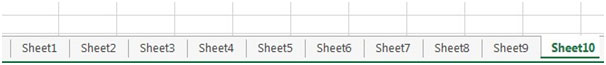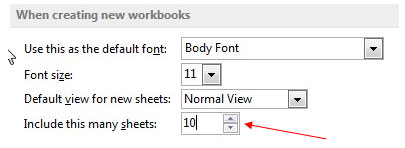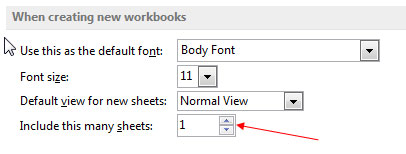Create new workbooks using VBA in Microsoft Excel

There could be instances where you need to create a new workbook with certain number of sheets through a vba code or macro. Here is a simple macro which allows you to create a new workbook > open & then save with a maximum of 255 new worksheets. In this sample macro, we will create a new workbook with 10 worksheets.

Option Explicit

Sub create_workbook()
Dim wb As Workbook
Set wb = NewWorkbook(10)
End Sub

Function NewWorkbook(wsCount As Integer) As Workbook
Dim OriginalWorksheetCount As Long
Set NewWorkbook = Nothing
If wsCount< 1 Or wsCount> 255 Then Exit Function
OriginalWorksheetCount = Application.SheetsInNewWorkbook
Application.SheetsInNewWorkbook = wsCount
Application.SheetsInNewWorkbook = OriginalWorksheetCount
End Function

Code Explanation:

First we have a procedure called “create_workbook”.  In this procedure, we have a variable wb as workbook and we call the function NewWorkbook through this variable. So the new function is called and the wscount is set at 10 which is the count of worksheets in the new workbook.

Then we go to the called function which is Function NewWorkbook(wsCount as Integer) as Workbook.  The value for wsCount which is 10 is passed on from the create_workbook sub.

Set NewWorkbook = Nothing

First the NewWorkbook is set to nothing so that we can clear out any other value which could be assigned to it in any prior codes.

If wsCount< 1 or wsCount> 255 then Exit Function

Next, we have to check if the value passed on from the sub is less than 1 or greater than 255.  If the answer is yes to either of these checks, then exit the function.
OriginalWorksheetCount = Application.SheetsInNewWorkbook

There is a parameter in the excel options page where the number of sheets to be included in a new workbook is set.  We pass this value to the variable OriginalWorksheetCount.The variable will hold the default number of sheets which was already pre-set in the excel options.

Application.SheetsInNewWorkbook = wsCount

Then we assign the value of wsCount which is passed on from the sub to the parameter in the excel options for the number of sheets to be included in a new workbook.  So now this parameter will change from the old value to 10.  The old value could be 1 or 3 or any other number set by the user.

We create a new workbook which is assigned to NewWorkbook.  This new workbook is created with the number of sheets specified in wsCount.  You will see a new workbook with 10 sheets titled Sheet1 to Sheet10.  See the pic below for the new file Book3 which has been created.The picture below will show you the number of sheets which were created in the new workbook.Also the number 10 will show up in the Application.SheetsInNewWorkbook parameter in Excel Options as in the picture below –Application.SheetsInNewWorkbook = OriginalWorksheetCount

TheApplication.SheetsInNewWorkbook is returned to its original number which was present before the wsCount was set. So if the original number was 1 or 3, this parameter will now return to that number.